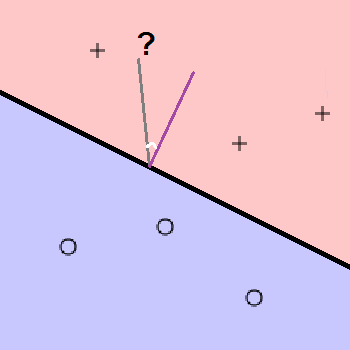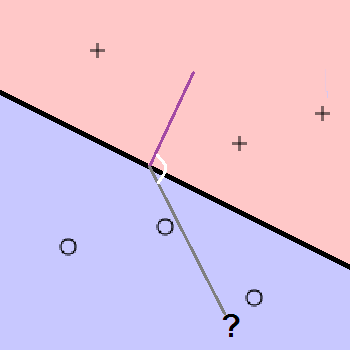# Perceptron Learning Algorithm

## Animation

Your browser does not support html5 canvas
Learning rate:

## The Perceptron Model

The perceptron is a simple but limited linear model. A perceptron separates space using a hyperplane (note: in two dimensions this is a line). Points above the plane are treated as being in the positive class while points below are treated as negative instances. This model is of limited use because most datasets with two classes are not linearly separable.

Any vector $$\Theta \in \mathbb{R}^{n + 1}$$ defines a hyperplane. This plane consists of all the points perpendicular to $$\Theta$$. For a perceptron classifier the decision boundary is a plane defined by such a vector. The direction of the vector determines the "up" side of the plane (ie: which side of the plane contains instances of the positive class).

Given a position vector $$x$$ that defines a point, we want determine which side of the plane it's on in order to classify it. To classify we look at the sign of $$\Theta^T x$$.

In $$\mathbb{R}^2$$ $$\Theta^T x = |\Theta||x|cos(\phi)$$ where $$\phi$$ is the angle between the vectors. In the case where $$x$$ is a positive example, $$\phi$$ is less than 90 degrees, so the dot product is positive. This case is illustrated by the following images where the thick line is the decision boundary, the purple line is $$\Theta$$, the grey line points to $$x$$, and $$\phi$$ is the white angle. Note that $$\Theta$$ is perpendicular to the boundary.In the case of a negative sample point, $$x$$ and $$\Theta$$ point in opposing directions so $$\phi$$ is between 90 and 180 degrees. This means the dot product is negative. Note that just as in the previous case, the sign of the dot product matches the point's class.